2.1
Algebra

Algebra is a branch of mathematics which deals with known values substituted for unknowns

Do you have interest in finding solutions to problems similar to:

1.     Find 9.5*10.5 quickly

2.     If the difference between 2 numbers is 8 and the difference between their squares is 400, What are those numbers? (Lilavati Shloka 59)

3.     Sum of my age and my father’s age is 55 years. If after 16 years, my father’s age is twice as that of mine, then can you tell me my age as on today?

4.     Suppose you along with your friends had planned a picnic. You had budgeted Rs.480 for food. But at the last moment 8 of your friends did not go for the picnic. Because of their absence other members paid Rs.10 extra for food. Find out how much each one paid finally?

5.     A plane left 30 minutes later than the scheduled time. In order to reach the destination 1500 km away it has to increase the speed by 250km/hr from its regular speed. Find the regular speed and its normal journey time.

We are solving these problems with the help of algebra in 2.4, 2.8, 2.14, 2.19 respectively.

2.1 Introduction to Algebra:

A Constant is a number whose value does not change. Examples are -4,0,1/3,5/2,1.19,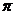A Variable is the one which does not have a fixed value and can take any value. Variables are represented by alphabets. The examples are x, y, a+b. They may take numerical values depending upon the problem

An Algebraic term is a number, a variable or the product of number and variables.

Examples are 4ab, 2x, 3y, 10, z, m/n, -p/q…

Let us look at the term 2x= 2*x which is the product of 2 and x. We call 2 as the numerical co-efficient of 2x. Similarly x is called literal factor or literal co-efficient of 2x.Terms having same literal factors with same exponents are called like terms.

Examples are

( x,2x, -7x)                --->(These have x as same literal factor)

(2mn, 5mn,-1/3mn)     --->( These have mn as same literal factor)

(x3, 5 x3, -5/6 x3)        --->(These  have x3 as the same literal factor). In these examples 3 is exponent (power) of x

Terms having different literal factors or same literal factors with different exponents are called unlike terms

Examples are:

( x, x3, x2)                --->(Though all have same x as literal factor the exponents are 1, 2 and 3 which are not same)

( 2x, 2a,-2mn)          --->( These  have x, a and mn as different literal factors)

An algebraic expression is a combination of constants and variables. (We can say it is combination of like and or unlike terms). Examples are

4x+ax3+9x2+ (2a/3b), -2mn+45+ y-2+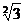+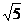A ‘polynomial’  is an algebraic expression in which variables have only positive integral exponents. Examples are:

4x+ax3+9x2+(2a/3b), -2mn+45

Note   y-2+ x3/2  is not a polynomial because  y has – 2 as exponent and  x  has  3/2 as exponent which are  not positive integers.

 Types of  Algebraic expression Examples An algebraic expression is called monomial if it has one term 3a, 2,-1/3y, An algebraic expression is called binomial if it has two terms 3-4a, 5x2-z An algebraic expression is called trinomial  if it has three terms 4x+ax3+9x2

A polynomial is said to be in standard form if its terms are in ascending/descending powers of the variables:

Example :

The term y2-2y4+3y-y3+4 is in non standard form.

Same term is rewritten in the standard form as

-2y4-y3+ y2+3y+4  or 4+3y+ y2-y3-2y4

If the number of variables in the polynomial is ‘n’ then it is called a polynomial in ‘n’ variables.

Examples :

1. 9x5+3x3+9x2+7x+5 is polynomial in one variable(x is the only variable)

2. 9x5+ax3+9x2+7x+5 is polynomial in two variables(x and a are the 2 variables)

The highest of sum of exponents of  all variables in a polynomial is called the degree of the polynomial.

Note: If p(x) is a polynomial of degree m and q(x) is a polynomial of degree n then the product p(x)*q(x) is a polynomial of degree m+n.

Examples:

1. The degree of 4y2- x2y2+ x2+6y is 4 (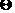the sum of exponents of x2y2 is 4(=2+2) which is the highest compared to the exponents of other terms 4y2, x2, 6y   which are 2, 2 and 1  respectively)

2. The degree of 10p3q2+4p2q-5+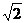p4 is 5 (the sum of exponents of p3q2 is 5(=3+2) which is the highest compared to the exponents of other terms 4p2q ,p4  which are 3(=2+1)  and 4  respectively)

The rules and properties (associative, distributive) applicable for arithmetic operations also apply for arithmetic operations involved with variables

For example we know 5 – (-6) = 5+6 =11:  -2 – (+5) = -2-5 = -7 and so on. Similarly (a+b)+c =a+(b+c) …….

In the case of algebraic expressions, while adding like terms, their numerical co-efficients are added:

For example:

1)8y4 -2y4=(8-2)y4=6y4

2) -11ab +-6ab = {-11+(-6)}ab = (-11-6)ab = -17ab

Unlike terms can not be added.

For example 8y4 -2y2 can not be further added and simplified

2.1.Problem 1:  Add  5a2-6a+3, 2a2+3a-1, 3a2-a-5

Solution :

The problem can be written as

(5a2-6a+3)+ (2a2+3a-1) + (3a2-a-5)

=5a2-6a+3+2a2+3a-1+3a2-a-5

= (5a2+2a2 +3a2) + (-6a+3a-a) + (3-1-5) (By grouping like terms to be together)

= (5+2+3) a2 + (-6+3-1)a + (3-1-5) (By adding the co efficients  of like terms)

=10 a2 + (-4a)-3

=10a2 -4a-3

2.1.Problem 2: Subtract 2x3-x2+4x-6 from x3+5x2-4x+6

Solution :

The problem can be written as

(x3+5x2-4x+6) – (2x3-x2+4x-6)

= x3+5x2-4x+6 - 2x3 -(-x2) -(+4x) –(-6)

=x3+5x2-4x+6 - 2x3+x2-4x+6 (-(- x2) = x2 and –(-6) =+6 )

= (x3 - 2x3)+(5x2+x2)+(-4x -4x) +(6+6) (By grouping like terms to be together)

= - x3+6x2-8x +12

2.1.Problem 3 : What must be subtracted from x3+2x2-3x+7 to get  x3+x2+x -1 ?

Solution :

The problem is similar to ‘what must be subtracted from 9 to get 3? The answer we know is 6 and is got by 9-3(=6)

Similarly we need to find (x3+2x2-3x+7) – (x3+x2+x -1)

(x3+2x2-3x+7) – (x3+x2+x -1)

= x3+2x2-3x+7 – x3-x2-x –(-1)

= (x3– x3)+(2x2-x2)+(-3x –x) +(7+1) (–(-1) =+1 )

= 0+x2-4x+8

= x2-4x+8

In this example x3+2x2-3x+7 is minuend (x3+x2+x -1) is subtrahend   and   x2-4x+8 is the difference

Verification:

We know that

Minuend = subtrahend + difference

(x3+x2+x -1) + (x2-4x+8)

= x3+(x2 + x2) +(x-4x) -1+8 (By grouping like terms to be together)

= x3+2x2-3x -7 which is minuend given in the problem

2.1 Summary of learning

 No Points studied 1 Definitions of  Constants, variables, Degree, Monomial, Binomial, Trinomial, Polynomial